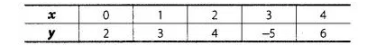# The coordinates of points in the table`
Question:

The coordinates of points in the tablerepresent some of the solutions of the equation x – y + 2 = 0.

Solution:

False

The coordinates of points are (0, 2), (1,3), (2, 4), (3, – 5) and (4, 6).

Given equation is x-y+2 = 0

At point (0,2), 0 – 2 + 2 = 0 => 0=0, it satisfies.

At point (1,3), 1-3+2 = 3-3 = 0 => 0 = 0, it satisfies.

At point (2, 4), 2-4+2 = 4- 4 = 0 => 0 = 0, it satisfies.

At point (3,-5), 3 – (- 5) + 2 = 3 + 5 + 2 = 10 ≠ 0, it does not satisfy.

At point (4, 6), 4-6+2-6-6 = 0 => 0 = 0, it satisfies.

Hence, point (3, – 5) does not satisfy the equation.